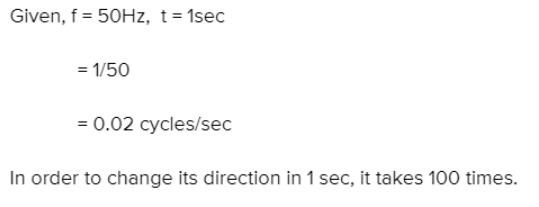Courses

# Test: Alternating Current (Competition Level 1)

## 30 Questions MCQ Test Physics Class 12 | Test: Alternating Current (Competition Level 1)

Description
This mock test of Test: Alternating Current (Competition Level 1) for Class 12 helps you for every Class 12 entrance exam. This contains 30 Multiple Choice Questions for Class 12 Test: Alternating Current (Competition Level 1) (mcq) to study with solutions a complete question bank. The solved questions answers in this Test: Alternating Current (Competition Level 1) quiz give you a good mix of easy questions and tough questions. Class 12 students definitely take this Test: Alternating Current (Competition Level 1) exercise for a better result in the exam. You can find other Test: Alternating Current (Competition Level 1) extra questions, long questions & short questions for Class 12 on EduRev as well by searching above.
QUESTION: 1

Solution:
QUESTION: 2

Solution:
QUESTION: 3

### The instantaneous value of current in an ac circuit is I = 2 sin (100pt + p/3) A. The current at the beginning (t = 0) will be

Solution:
QUESTION: 4

If instantaneous value of current is I = 10 sin (314 t) A, then the average current for the half cycle will be

Solution:
QUESTION: 5

In a circuit an a.c. current and a d, c. current are supplied together. The expression of the instantaneous current is given as i = 3 + 6 sin wt. Then the rms value of the current is

Solution:
QUESTION: 6

The emf and the current in a circuit are E = 12 sin (100pt) ; I = 4 sin (100pt + p / 3) then

Solution:
QUESTION: 7

If the frequency of alternating potential is 50Hz then the direction of potential, changes in one second by

Solution:QUESTION: 8

The value of alternating e.m.f. is e = 500 sin 100pt , then the frequency of this potential in Hz is

Solution:
QUESTION: 9

The domestic power supply is at 220 volt. The amplitude of emf will be

Solution:
QUESTION: 10

The average value or alternating current for half cycle in terms of I0 is

Solution:
QUESTION: 11

Sinusoidal peak potential is 200 volt with frequency 50Hz. It is represented by the equation

Solution:
QUESTION: 12

RMS value of ac i = i1 cos wt + i2 sin wt will be

Solution:
QUESTION: 13

The phase difference between the alternating current and voltage represented by the following equation I = I0 sin wt, E = E0 cos (wt + p / 3), will be

Solution:
QUESTION: 14

The inductive reactance of a coil is 1000W. If its self inductance and frequency both are increased two times then inductive reactance will be

Solution:
QUESTION: 15

In an L.C.R series circuit R = 1W, XL = 1000W and XC = 1000W. A source of 100 m.volt is connected in the circuit the current in the circuit is

Solution:
QUESTION: 16

A coil of inductance 0.1 H is connected to an alternating voltage generator of voltage E = 100 sin (100t) volt. The current flowing through the coil will be

Solution:
QUESTION: 17

Alternating current lead the applied e.m.f. by p/2 when the circuit consists of

Solution:
QUESTION: 18

A coil has reactance of 100W when frequency is 50Hz. If the frequency becomes 150Hz, then the reactance will be

Solution:
QUESTION: 19

A resistance of 50W, an inductance of 20/p henry and a capacitor of 5/p mF are connected in series with an A.C. source of 230 volt and 50Hz. The impedance of circuit is

Solution:
QUESTION: 20

The potential difference between the ends of a resistance R is VR between the ends of capacitor is VC = 2VR and between the ends of inductance is VL = 3VR, then the alternating potential of the source in terms of VR will be

Solution:
QUESTION: 21

The percentage increase in the impedance of an ac circuit, when its power factor changes form 0.866 to 0.5 is (Resistance constant)

Solution:
QUESTION: 22

In a series resonant L_C_R circuit, if L is increased by 25% and C is decreased by 20%, then the resonant frequency will

Solution:
QUESTION: 23

Which of the following statements is correct for L_C_R series combination in the condition of resonance

Solution:
QUESTION: 24

An ac circuit resonates at a frequency of 10 kHz. If its frequency is increased to 11 kHz, then

Solution:
QUESTION: 25

In an ac circuit emf and current are E = 5 cos wt volt and I = 2 sin wt ampere respectively. The average power dissipated in this circuit will be

Solution:
QUESTION: 26

A choke coil of negligible resistance carries 5 mA current when it is operated at 220 V. The loss of power in the choke coil is –

Solution:
QUESTION: 27

In an A.C. circuit, a resistance of 3W, an inductance coil of 4W and a condenser of 8W are connected in series with an A.C. source of 50 volt (R.M.S.). The average power loss in the circuit will be

Solution:
QUESTION: 28

Two bulbs of 500 watt and 300 watt work on 200 volt r.m.s. the ratio of their resistances will be –

Solution:
QUESTION: 29

Three bulbs of 40, 60 and 100 watt are connected in series with the source of 200 volt. Then which of the bulb will be glowing the most –

Solution:
QUESTION: 30

Alternating current can not be measured by direct current meters, because –

Solution: